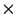Next: Production:VTX;Space_Point Up: Banks in the Event Previous: Generator:Header

## Generator:Particle_List


Element# 		: 		 Equal to the track number.
Contents 		: 		 Particles output by hadronizer, including stable and
unstable particles depending on the case.
Format 		: 		 R*4, 20 words fixed.



RBUF(1)=Particle serial number
(2)=Particle ID (PDG Format).
(3-4)=Particle mass (GeV) and charge.
(5-8)=Í<>px, py, pz, and E (GeV)
(9-11)=Vertex coordinate, x, y, z (cm).
(12)=Number  of daughter particle (=0 if stable. )
(13)=Pointer to the daughter
(14)=Pointer to the mother.  If LT 0, equal to the element
number of SPRING:Parton_List corresponding to this particle.
(15)=trigger timing(nsec).
(16)=particle life time (cnsec, =0. or negative for stable.)
(17)=decay length (cm, =0. for stable.)


Miyamoto Akiya
11/20/1998